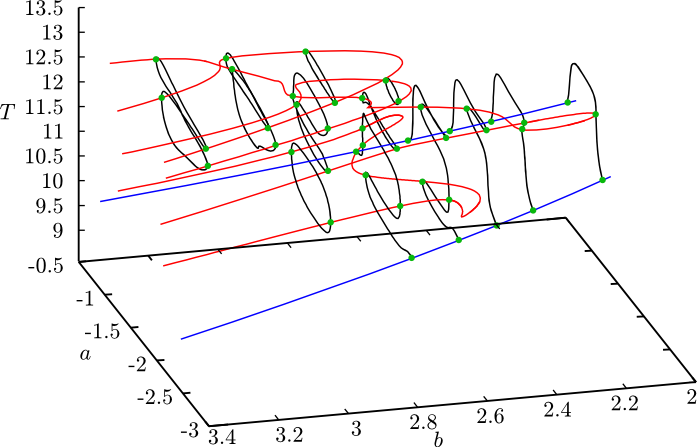# Knut

A numerical continuation software

View the Project on GitHub rs1909/knut

## Capabilities

The software is designed to analyse explicitly time-dependent delay-differential equations with time dependent delays of the form $\boldsymbol{M}\,\dot{\boldsymbol{x}}(t) = \boldsymbol{f} (t, \boldsymbol{x}(t-\tau_0(t)), \boldsymbol{x}(t - \tau_1(t)), \dots , \boldsymbol{x}(t - \tau_m(t)))$ The mass matrix $\boldsymbol{M}$ can be singular, hence Knut can handle algebraic equations and neutral delay-differential equations. State dependent delays are not yet supported, see DDE-BIFTOOL for that.

The features include

• Continuation of periodic orbits along a parameter
• Calculation of Floquet multipliers of periodic orbits
• Automatic detection of bifurcations
• Continuation of torus, period doubling and fold bifurcations of periodic orbits along two parameters
• Continuation of invariant tori with quasi-periodic orbits along two parameters
• Continuation of steady states (as periodic orbits)

Significant differences from DDE-BIFTOOL

• No need for any programming, equations entered using simple formulae
• Stand-alone software
• Graphical User Interface
• Written in C++, hence faster
• The only software that calculates quasi-periodic tori

The Graphical User Interface also includes a plotting tool that displays the calculation live.

### Sample equation specification

The Mackey-Glass equation $\dot{x} ( t ) = ax ( t ) + b \frac{x ( t - \tau )}{1 + x^{10} ( t - \tau )}$ is represented by the following code

 vfname()=mackey_glass; time()=t; period(T)=2.0; par(a)=-1.0; par(b)=1.5; par(tau)=2.0; DX=delay(X,tau); dot(X)=a*X + b*DX/(1+DX^10); init(X)=((1.0-1.5)/(-1.0))^(1.0/10.0);

### Bifurcation diagram of the Mackey-Glass equationFigure 1. Fold bifurcations of the period-two orbits. Black curves are periodic solutions continued along $b=$constant lines. Red curves refer to fold bifurcations, and the blue line is the period doubling curve, where the period-two orbits arise.

## Documentation

See the Users' manual

## Compilation instructions

Dependencies are OpenBLAS, CMake and optionally Qt

To build the software use the following commands:

 git clone https://github.com/rs1909/knut.git cd knut/knut mkdir build cd build ../build-unix.sh make install

On Mac OSX replace the penultimate command with ../build-mac.sh
To make an installable package use make install and make dmg which will produce a disk image

On Fedora Linux with the MinGW cross-compiler installed the ../build-win-cross.sh will compile the software for Windows. To make a package use makensis wininstaller.nsi.

Please report any bugs and wishes on the issue tracker, I'll try to address them as my time allows it.